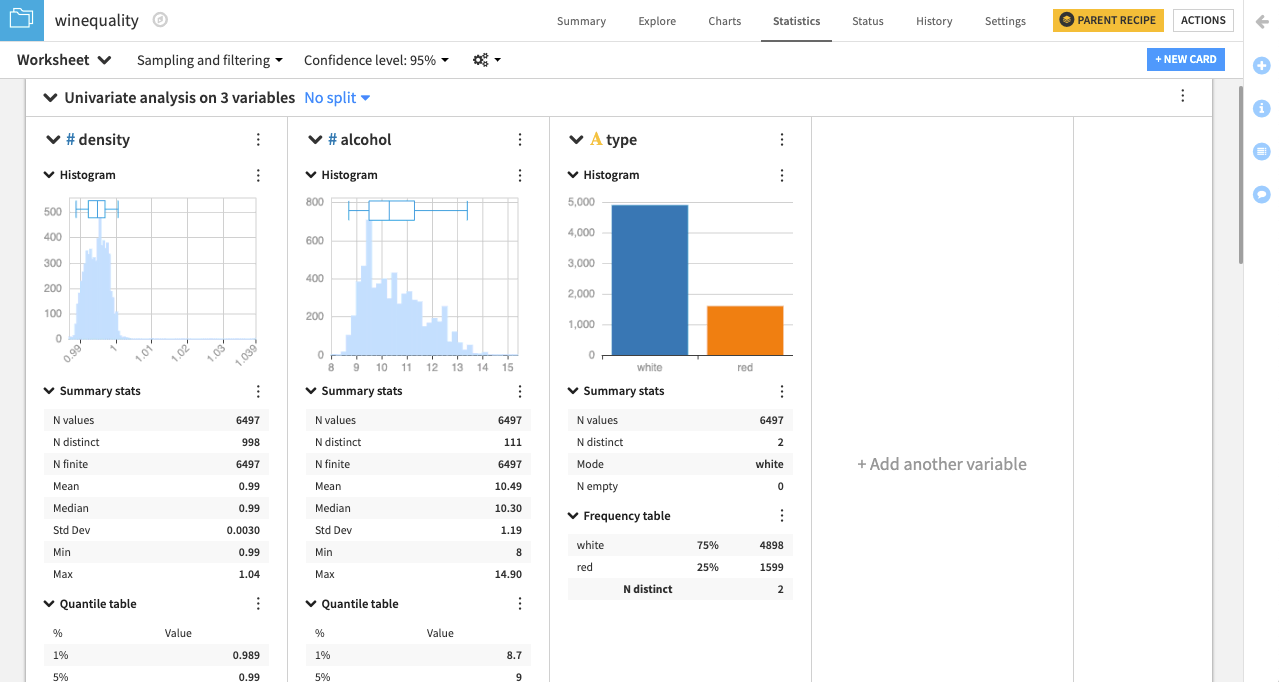# Tutorial | Perform univariate and bivariate analysis with a statistics card¶

Note

This lesson is a continuation of the Interactive Statistics hands-on tutorial.

## Univariate analysis¶

Univariate analysis is used to compare the data distribution of individual variables. Let’s use it to see a side-by-side comparison for the variables density, alcohol, and type.

Remember that the $$\boldsymbol{\#}$$ symbol denotes a numerical variable and the $$\mathrm{\mathbf{A}}$$ denotes a categorical variable.

• From the Select a card type window, click the Univariate analysis box. This brings up the Univariate analysis window.

• The first column of the window lists the number of available variables. Select density, alcohol, and type.

• Click the plus icon to add them to Variables to describe. You can also drag and drop the variables into Variables to describe if you prefer.

• Click Create Card to create the univariate analysis card.Note

Notice that Dataiku automatically selects the statistical Options to the right that are appropriate for the numerical variables (density and alcohol) and the categorical variable (type). You can deselect any of these options if needed.

Dataiku creates a card with one section for each variable. The type of statistical chart and descriptive statistic in each section depends on whether the variable is categorical or numerical.

In this case, the categorical variable type displays a categorical histogram, while density and alcohol each display a numerical histogram and box plot insert. Also, a quantile table is applied to the numerical variables, while a frequency table is applied to the categorical variable.Note

By default, Dataiku computes worksheet statistics on a sample of the first records in your dataset. You can configure this setting by clicking the dropdown arrow next to Sampling and filtering.

## Bivariate analysis¶

Bivariate analysis lets us examine the data distribution for pairs of variables simultaneously. In this section, we will examine the response variable (type) for each factor variable (density and alcohol).

• Click the New Card button from the Worksheet header and select Bivariate analysis.

• Add density and alcohol to the Factor(s) box.

• Add type to the Response box.

• Click Create Card to create the bivariate analysis card. Dataiku will create a card with one section for each factor-response pair.Notice that each descriptive statistical option (e.g. histogram) in the card has a pencil icon that appears when you hover over it that lets you choose additional configurations. For example, clicking the pencil for a histogram plot enables you to select a binning mode and maximum number of bins.

• To get a better view of the distributions from the histogram plots, click the pencil icon next to the density histogram.

• Set the density binning mode to Fixed nb. of bins.

• Set that number to be 100.

• Repeat the same for the alcohol histogram.Note

For more information, see Univariate Analysis and Bivariate Analysis in the reference documentation.